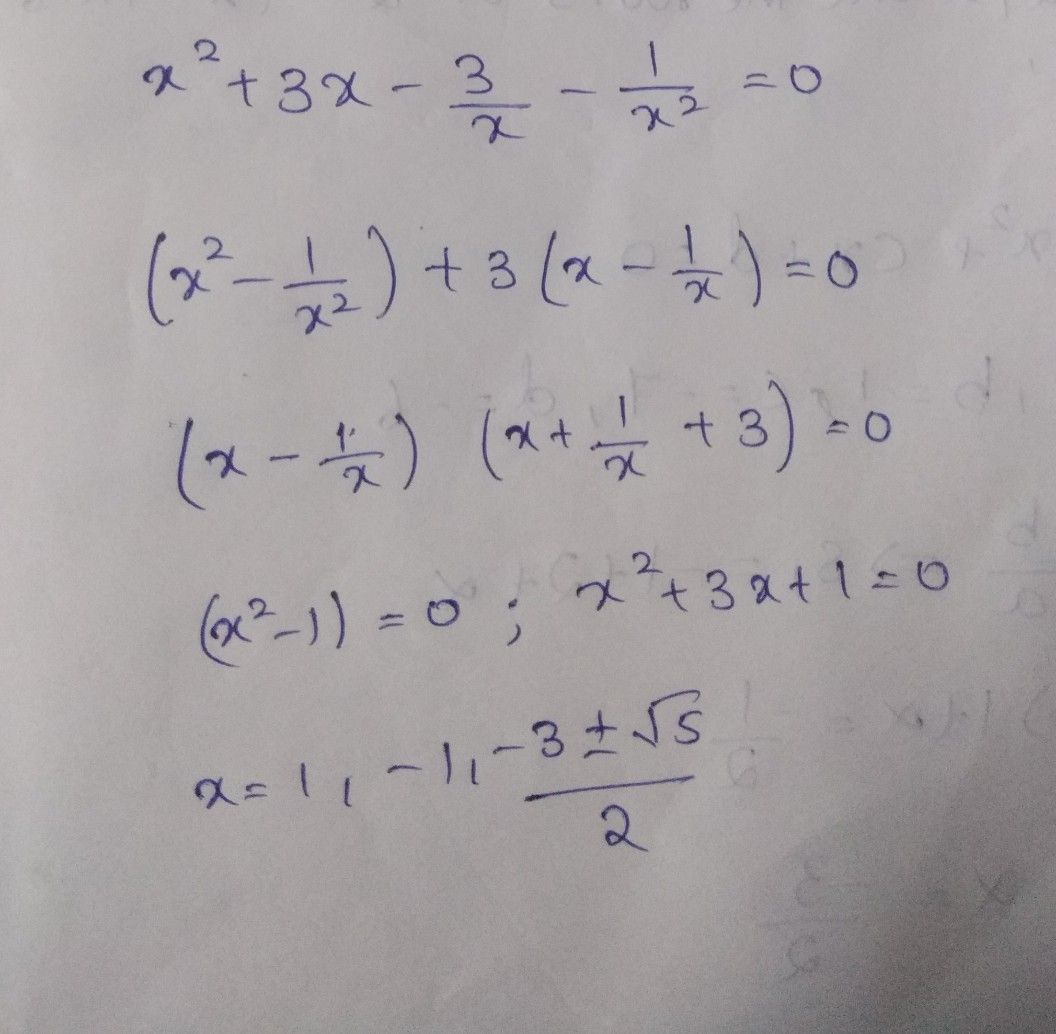Symbol
Problem$15.$ $z=1+i\sqrt{3} →|Argz|+|Arg\bar{z} |=$ $EAMCET2010$ $1\right)0$ $2\right)$ $\dfrac {\pi } {3}$ $3\right)$ $\dfrac {\pi } {2}$ $4\right)\dfrac {2\pi } {3}$
Algebra
SolutionQanda teacher - A Reddy